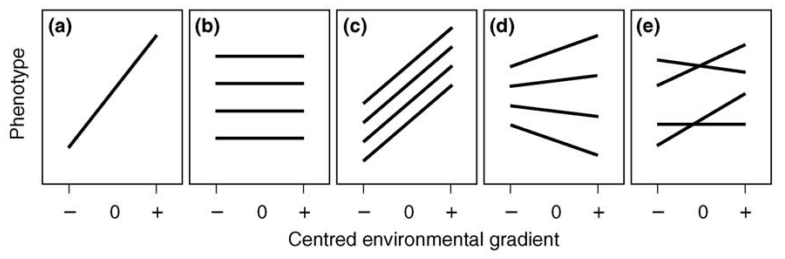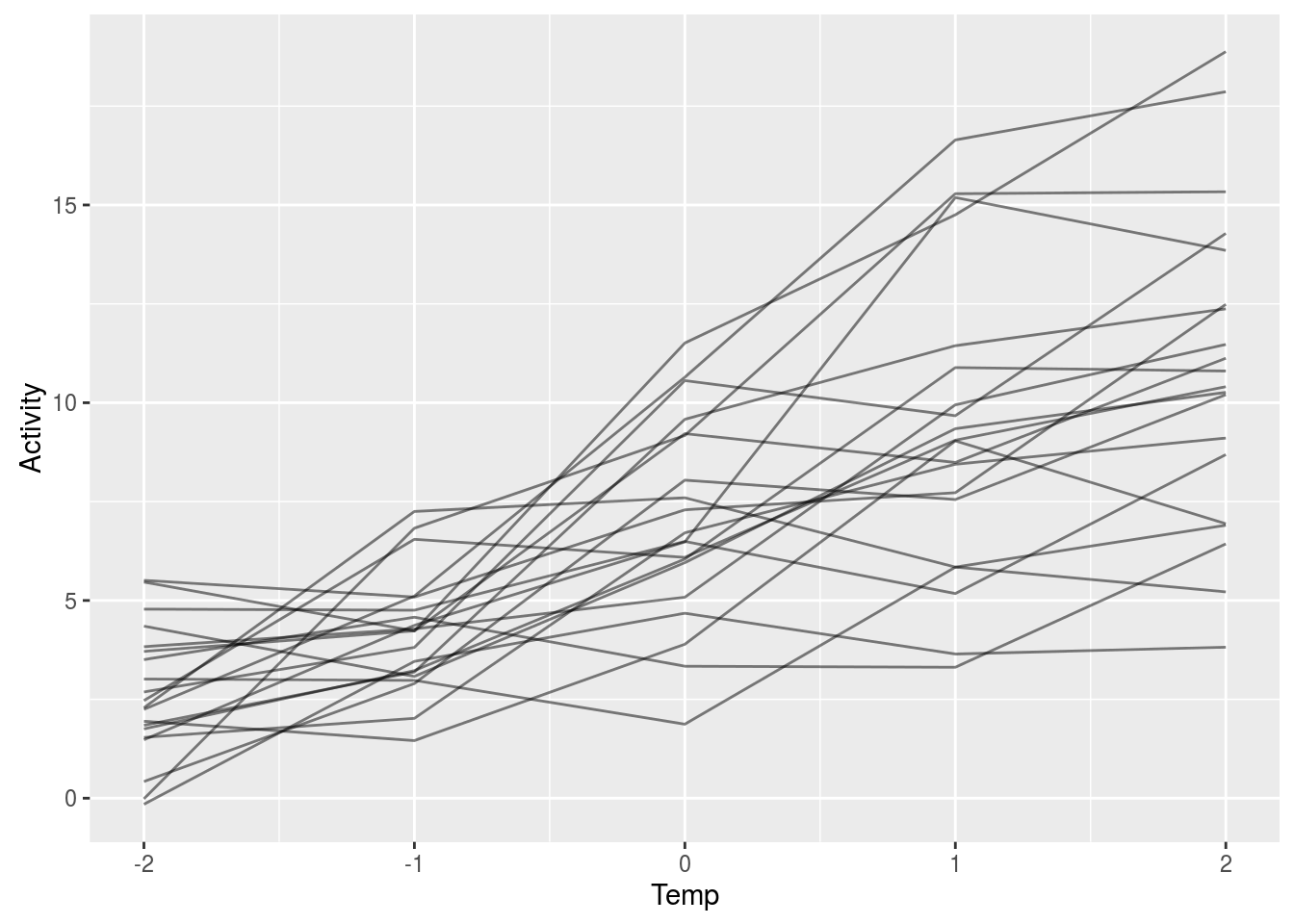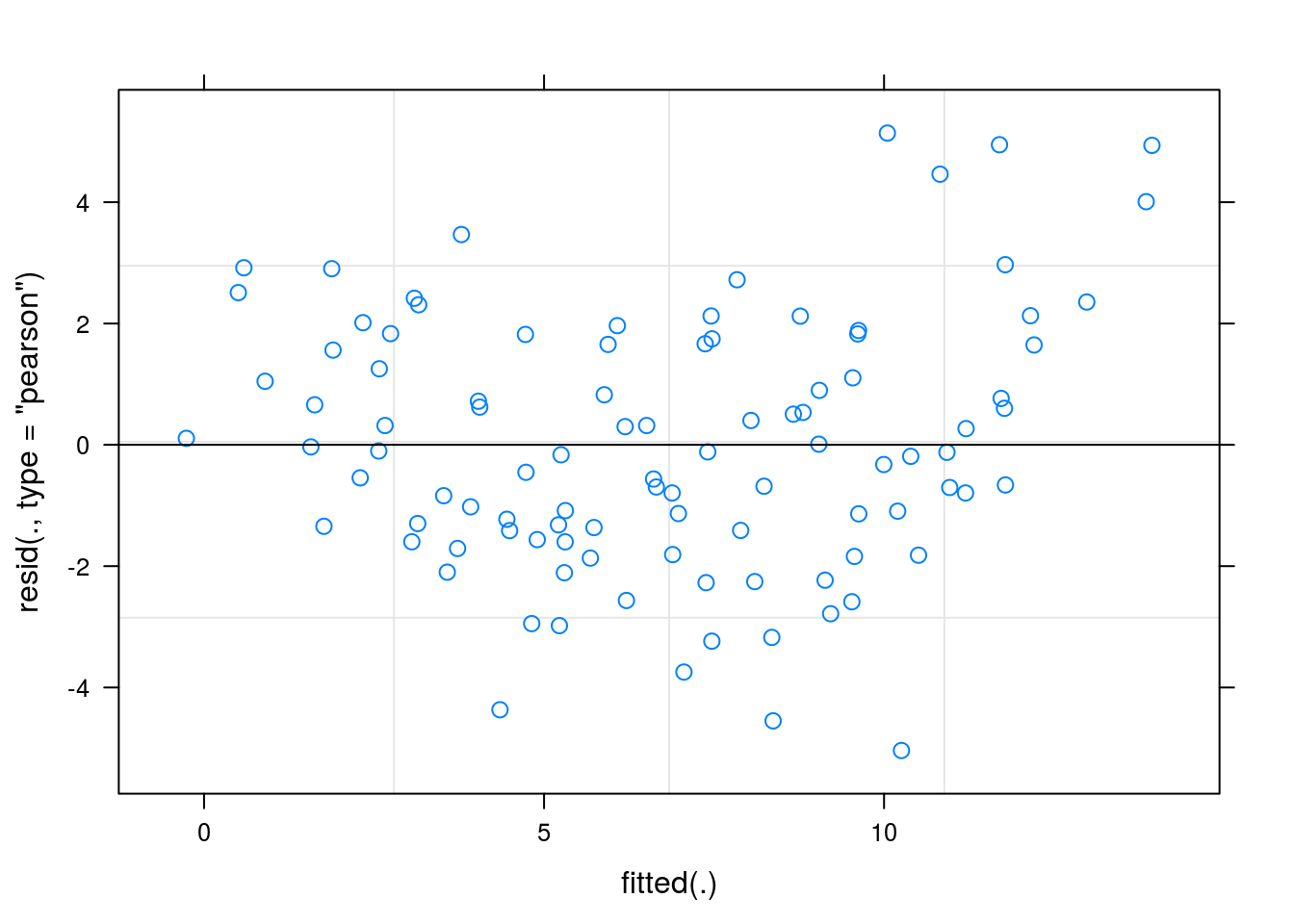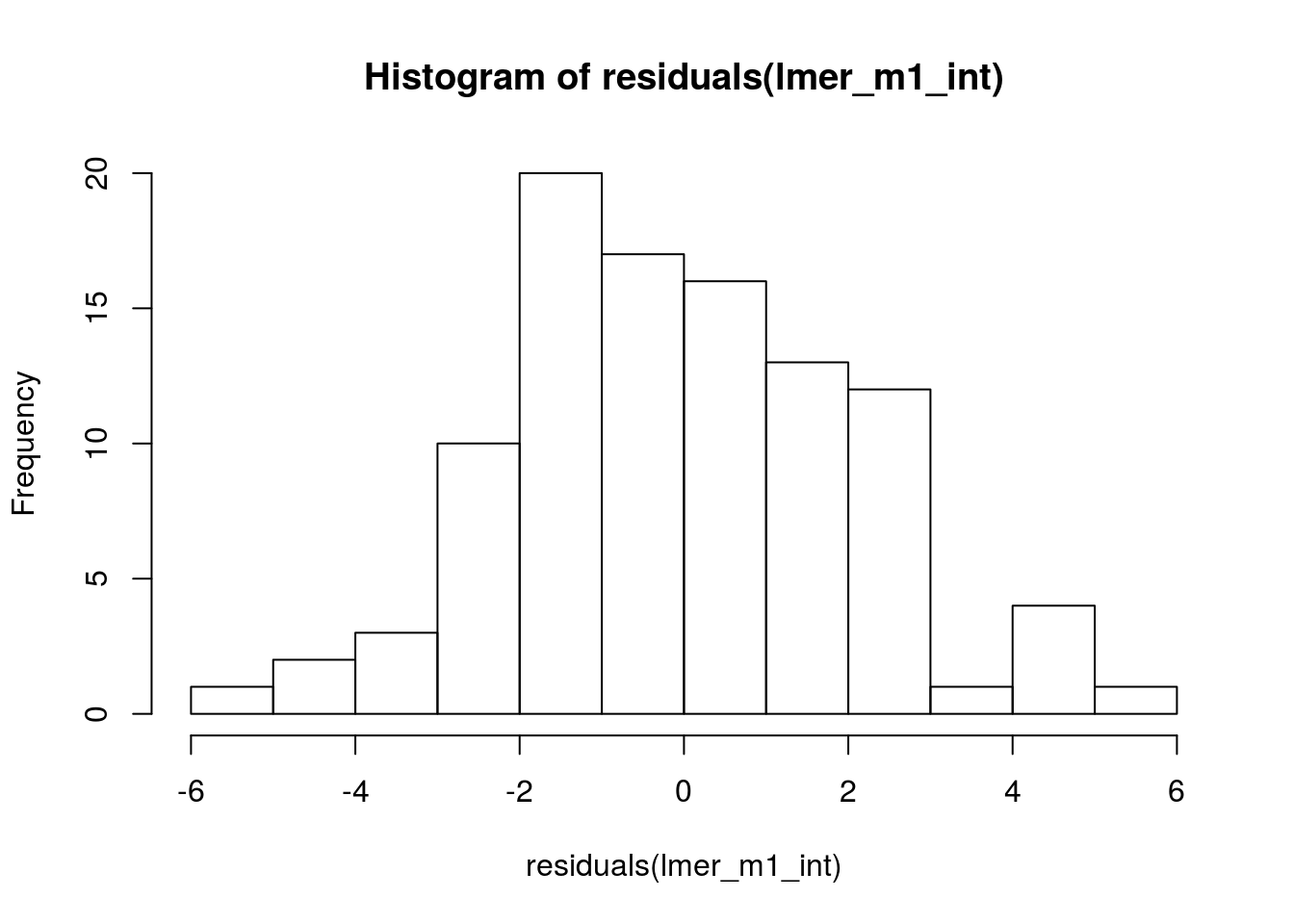## Introduction

This is a very quick tutorial to running models in R for plasticity (particularly among-individual differences in plasticity), created for Suzanne Alonso and Nick Royle’s ISBE symposium on behavioural plasticity. I am assuming some experience with regression models, but include brief pointers on specifying, performing diagnostics, and checking whether the model is a good fit. If you want to read more about the intricacies of random-intercept and -slope models, the University of Bristol’s Centre for Multi-level Modelling has some great material:

http://www.bristol.ac.uk/cmm/learning/videos/

If you find this useful, or have any comments / suggestions, you can email me at houslay@gmail.com.

There are a bunch of libraries to load (and install, if necessary) - not all of them are for actually running the models, but they’ll make life easier!

``````require(knitr)
require(tidyr)
require(dplyr)
require(ggplot2)
require(broom)
require(gridExtra)

require(lme4)

# lme4 and tidyr both have 'expand' functions -
#  we want to make sure it's tidyr's that we use when we call it!
expand <- tidyr::expand

# Function for standard error
std_err <- function(x) sd(x)/sqrt(length(x))``````

## Reaction norms approach

The most common approach to modelling variation in individual plasticity is the reaction norm approach - using random regression models to test for differences in individual slope deviations. This approach has been covered fairly extensively in the literature, e.g. Dingemanse et al 2010.Figure taken from Dingemanse et al (2010)

## Example overview

In this example, we have observed individual behaviour (‘Activity’) at 5 different temperatures, and want to test whether (i) there exists population-level plasticity, such that average activity is significantly affected by temperature, and (ii) there are individual differences in plasticity, such that slope deviations are significantly different from one another.

### Load and check simulated data

Next, we’ll load up some simulated data. This has 3 columns:

• ID (individual identifier)
• Temp (predictor variable: the temperature, in C, at which measurement was taken)
• Activity (the response variable: activity level, on some arbitrary scale)
``````df_activity <- data_frame(ID = factor(rep(LETTERS[1:20], each=5)),
Temp = rep(-2:2, times=20),
Activity = c(5.509483369,
5.086400559,
7.291669795,
7.7255394,
12.48731752,
-0.15231211,
3.459667228,
4.676662498,
3.646438077,
3.819056263,
1.845028096,
3.191492183,
9.580782097,
11.4420279,
12.37367193,
4.782295151,
4.754364267,
6.493168431,
5.175259749,
8.687935843,
-0.014547574,
6.828039886,
9.172530306,
15.28585964,
15.33542768,
3.83089209,
4.281952234,
5.08286246,
9.947459161,
11.47400038,
2.68590715,
3.814660944,
10.56006869,
9.671785115,
14.28151694,
5.465825545,
4.22941294,
9.21819825,
8.490583698,
11.12413906,
3.5050106,
4.576850562,
3.337903469,
3.312161935,
6.430644692,
4.350695078,
3.080603398,
5.956493065,
9.342901914,
10.26237114,
2.468397656,
6.546708098,
6.090473345,
9.052316868,
10.40528841,
0.420737959,
2.899078212,
8.042977408,
7.552856261,
10.20289061,
2.287388649,
7.251450611,
7.597332849,
5.844943899,
5.217627539,
2.246044457,
5.111583578,
10.64544808,
16.64489096,
17.8635928,
3.014025209,
2.979938777,
1.872008574,
5.843502353,
6.902012099,
1.537929061,
2.020490233,
6.711361941,
8.444715807,
9.106907328,
1.478295133,
4.370893504,
6.481001168,
15.18832065,
13.85280053,
3.711452311,
4.231790222,
11.51008315,
14.75226205,
18.87686314,
1.946090462,
1.457254528,
3.893480056,
9.035952938,
6.940550594,
1.752620681,
3.227335752,
6.047545853,
10.88963854,
10.80254743))``````

Take a quick look at the structure of the data:

``str(df_activity)``
``````## Classes 'tbl_df', 'tbl' and 'data.frame':    100 obs. of  3 variables:
##  \$ ID      : Factor w/ 20 levels "A","B","C","D",..: 1 1 1 1 1 2 2 2 2 2 ...
##  \$ Temp    : int  -2 -1 0 1 2 -2 -1 0 1 2 ...
##  \$ Activity: num  5.51 5.09 7.29 7.73 12.49 ...``````
``head(df_activity)``
``````## # A tibble: 6 x 3
##       ID  Temp   Activity
##   <fctr> <int>      <dbl>
## 1      A    -2  5.5094834
## 2      A    -1  5.0864006
## 3      A     0  7.2916698
## 4      A     1  7.7255394
## 5      A     2 12.4873175
## 6      B    -2 -0.1523121``````

We can see that our data structure is ‘tidy’; it maps how we want to think about and use the data (each row is a single observation of a single individual). We can use ggplot to plot the data with individual lines:

``````ggplot(df_activity, aes(x = Temp, y = Activity, group = ID)) +
geom_line(alpha = 0.5)``````### Random intercept model

We will first model our data using a mixed model with ‘random intercepts’ only - we want to include a fixed effect of ‘Temp’ (population-level plasticity), and allow individuals to have distinct intercepts:

``````lmer_m1_int <- lmer(Activity ~ Temp + (1|ID),
data = df_activity)

plot(lmer_m1_int)````````hist(residuals(lmer_m1_int))````qqnorm(residuals(lmer_m1_int))``